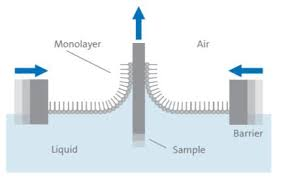The study of physisorption goes back nearly 200 years and aims to explain the science of gas interactions on the surface of a material. This gas is known as an adsorbate and the process of this interaction is called adsorption. One of the many groundbreaking scientists who worked in this field in the 18th century was Irving Langmuir, whose findings became the foundation for a plethora of additional adsorption studies commonly used today.

Langmuir started his studies in metallurgical engineering before turning his attention to physical chemistry, where he sought to understand how atoms and molecules reacted to being in high vacuum environments. In 1916, the Langmuir film balance was created. This consisted of a trough of liquid with a vertical sample plate in the middle. Barrier plates on either side of the trough could be shifted to change the surface area and increase the pressure of the liquid on the sample plate. An animation of the process can be seen at https://www.youtube.com/watch?v=j8yqyRr2VQg. It was found that a single layer of molecules had deposited on the surface. This was dubbed a monolayer.From here, Langmuir made assumptions that “layers of gasses adsorbed on solid surfaces possess as unimolecular nature.” and derived his adsorption isotherm equation. He published his work which earned him a Nobel Prize in 1932 for surface chemistry. His work included the following equation:$\theta=\frac{KP}{1+KP}=\frac{r_{inc}p_{ads}}{r_{des,sat}+r_{inc}p_{ads}}$

•$\theta$ is the equilibrium fractional occupancy, meaning the fraction of active sites that are occupied at equilibrium as compared to the total number of active sites.
• active sites are locations on the surface that are available to interact with a molecule of the gas adsorbate
• K is the langmuir constant
• P is the pressure of the gas phase
•$r_{inc}$ is the rate of incidence, which is proportional to the pressure at constant temperature using the kinetic theory of gasses
•$p_{ads}$ is the probability of gas adsorption
•$r_{des,sat}$ is the rate of desorption when the surface is fully covered

The Langmuir adsorption model is most common for quantifying the amount of adsorbate adsorbed onto a material (called an adsorbent) as a function of partial pressure at a given temperature. It includes the following assumptions:

1. The surface of the sample (adsorbent) only contains a certain number of active sites, at which only one molecule may be adsorbed.
2. There is no lateral interaction between the adsorbed molecules, meaning that gas molecules adsorbed close to one another don’t cause any alteration to each other.
3. The adsorption is localized, i.e. the adsorbed molecule stays on the surface until it is desorbed.
4. Only a single layer of molecules is formed.

Because of these assumptions, the Langmuir adsorption model is best used to determine surface area, though it fails to account for surface roughness (which causes lateral interactions). It is best applied to samples with smooth surfaces. It also only accounts for the adsorbed monolayer, so many modifications have been made to the model over time to make it more suitable for a wider range of sample types. The Freundlich and Temkin models are examples of such.

Langmuir reports and models are one of the many options available on the Micromeritics 3Flex in the lab.

References: